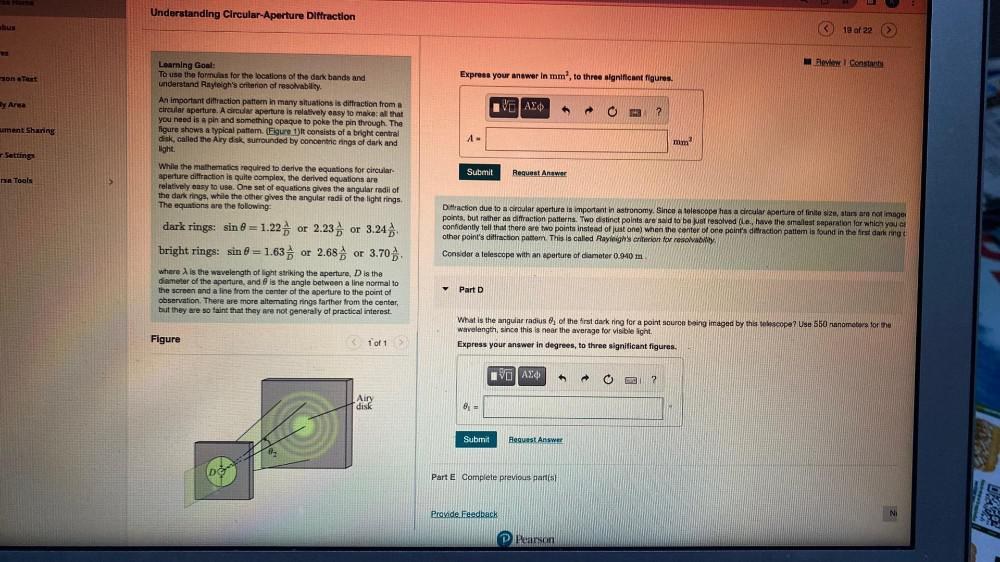Question:

# home Understanding Circular-Aperture Diffraction bus 19 of 22 es Review Constants Express your answer in mm", to three significahome Understanding Circular-Aperture Diffraction bus 19 of 22 es Review Constants Express your answer in mm", to three significant figures. son eText hy Area Learning Goal: To use the formulas for the locations of the dark bands and understand Rayleigh's criterion of resolvability. An important dittraction pattem in many situations is diffraction from a circular aperture. A circular aperture is relatively easy to make: all that you need is a pin and something opaque to poke the pin through. The figure shows a typical pattem. (Figure 1)It consists of a bright central disk, called the Airy disk, surrounded by concentric rings of dark and light. VO AED ? ument Sharing A = mm2 Settings Submit Request Answer rse Tools While the mathematics required to derive the equations for circular- aperture diffraction is quite complex, the derived equations are relatively easy to use. One set of equations gives the angular radil of the dark rings, while the other gives the angular radii of the light rings. The equations are the following: Diffraction due to a circular aperture is important in astronomy. Since a telescope has a circular aperture of finite size, stars are not images points, but rather as diffraction patterns. Two distinct points are said to be just resolved (i.e., have the smallest separation for which you ca confidently tell that there are two points instead of just one) when the center of one point's diffraction pattern is found in the first dark ring other point's diffraction pattern. This is called Rayleigh's criterion for resolvability, Consider a telescope with an aperture of diameter 0.940 m dark rings: sin 0 = 1.22 or 2.23 or 3.24 bright rings: sin 8 = 1.63 or 2.689 or 3.70 where ) is the wavelength of light striking the aperture, D is the diameter of the aperture, and is the angle between a line normal to the screen and a line from the center of the aperture to the point of observation. There are more alternating rings farther from the center, but they are so faint that they are not generally of practical interest. Part D What is the angular radius of the first dark ring for a point source being imaged by this telescope? Use 550 nanometers for the wavelength, since this is near the average for visible light. Express your answer in degrees, to three significant figures. Figure 1 of 1 VO AEO ? Airy disk @1 = Submit Request Answer 02 DO Part E Complete previous part(s) O 02 Provide Feedback N Pearson